# The Prisoner of Benda and the Futurama Theorem

## Futurama

• Futurama is an American animated science fiction sitcom created by Matt Groening (of The Simpsons).
• The series aired on Fox from 1999-2003, and then began airing on Comedy Central in 2008. Season 7 coming in 2012.
• The show follows the adventures of a late 20th-century New York City pizza delivery boy, Philip J. Fry, who was accidentally frozen in 1999 and then thawed out on New Year's Eve 2999.
• Some main characters: Fry, Leela (one-eyed alien), Bender (robot), Professor Farnsworth (Fry's distant nephew and mad scientist), Zoiberg (lobster-like alien), Amy (spoiled and accident prone student), and more.

## Futurama

Here is short clip with the Professor Farnsworth and the Globe Trotters:

## The Prisoner of Benda

• "The Prisoner of Benda" is episode 10 of season 6 and aired on August, 2010.
• The title is a play on "The Prisoner of Zenda", a 1894 novel by Anthony Hope Hawkins.
• In the episode, Farnsworth and Amy build a machine that allows them to switch minds. However, they learn that the machine cannot be used twice on the same pair. After several characters swap minds, the rest of the episode is focused on how to return everyone's minds back to their original owner.
• With the help of two Harlem Globetrotters (and some mathematics), the irreversible mind swap problem is solved.
• Episode written by Ken Keeler, who has a PhD in applied mathematics from Harvard.
• Keeler invented and proved a mathematical theorem specifically for the show.

## The mind swap problem

Here are the swaps that take place during the episode:

• Professor ($p$) $\leftrightarrow$ Amy ($a$)
• Amy ($a$) $\leftrightarrow$ Bender ($b$)
• Professor ($p$) $\leftrightarrow$ Leela ($l$)
• Amy ($a$) $\leftrightarrow$ Wash Bucket ($w$)
• Fry ($f$) $\leftrightarrow$ Zoiberg ($z$)
• Emperor Nikolai ($e$) $\leftrightarrow$ Wash Bucket ($w$)
• Hermes($h$)$\leftrightarrow$ Leela ($l$)

The upshot: $a \rightarrow h \rightarrow l \rightarrow p \rightarrow b \rightarrow e \rightarrow w \rightarrow a \text{ and } f \leftrightarrow z$

## The mind swap problem (continued)

The question is:

Can the machine be used to put everyone back if we aren't allowed to use the machine on the same pair of bodies more than once?

## We need to use math!

In the first 25 seconds of this video, we see that the Professor, Bender, and Amy realize that they are in trouble.

Note:This video was made by SingingBanana, and if you watch the whole video, you'll get another full description of the problem and solution.

## The symmetric group

We can model this problem in terms of permutations (i.e., rearrangements) of numbers. It turns out that the set of all permutations of the numbers 1 through $n$ forms a "group" under composition.

A group is a set with an associative binary operation satisfying:

• #### closure:

the "product" of any two elements from the set is an element of the set.
• #### identity:

there exists a "do nothing" element.
• #### inverses:

for every element in the set, there exists another element in the set that "undoes" the original.

The group of permutations of $\{1,2,\ldots, n\}$ is called the symmetric group and is denoted by $S_n$.

## Permutation diagrams

One way of representing an element of the symmetric group is via permutation diagrams, which we illustrate by way of example.

### Example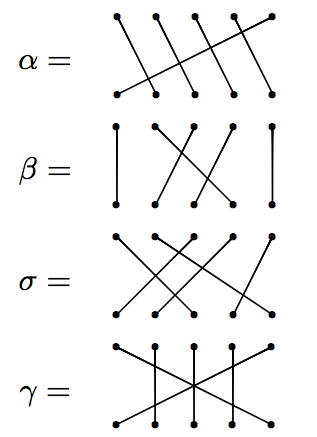## Permutation diagrams (continued)

Let's try multiplying.

### Example

Let $\alpha$ and $\beta$ be as on previous slide. Then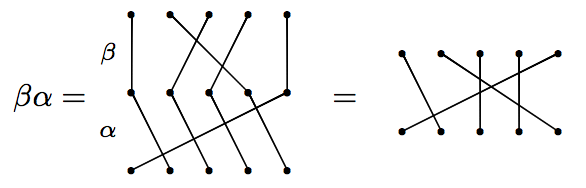But on the other hand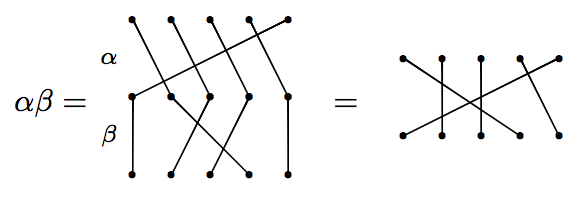We see that products of permutations do not necessarily commute (order matters).

## Permutation diagrams (continued)

However, sometimes permutations do commute.

### Example

Let $\beta$ and $\gamma$ be as before. Then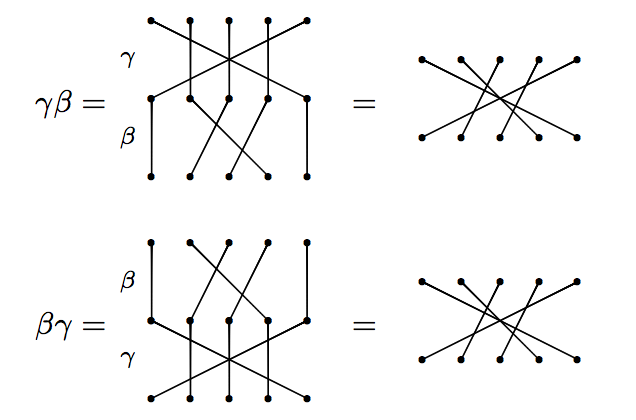Notice that these result in the same diagram. So, $\beta$ and $\gamma$ commute.

## Permutation diagrams (continued)

Let's do one more example.

### Example

Let $\gamma$ be as before. Then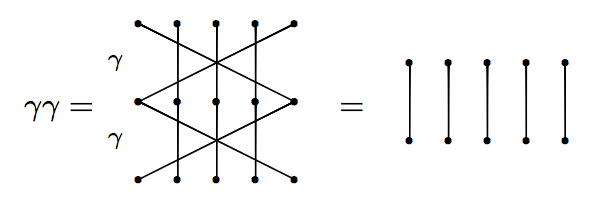The rightmost diagram is the identity in $S_{5}$ (it's the "do nothing action"). Since $\gamma \gamma$ is equal to the identity, $\gamma$ must be its own inverse.

• Order matters, but not always.
• The inverse of a permutation is just the vertical flip of the corresponding diagrams.
• A diagram that swaps precisely two numbers is called a transposition.
• Every transposition is its own inverse.
• "Disjoint" transpositions commute.
• $S_n$ has $n!$ many elements.
• $S_n$ is generated by the transpositions. That is, every permutation can be written as a product of some sequence of transpositions. In fact, we only need the adjacent transpositions: $i$ swaps with $i+1$.

## The mind swap problem as diagrams

If we let $a=1$, $h=2$, $l=3$, $p=4$, $b=5$, $e=6$, and $w=7$, $f=8$, and $z=9$, then the mind swap problem from Futurama can be depicted as: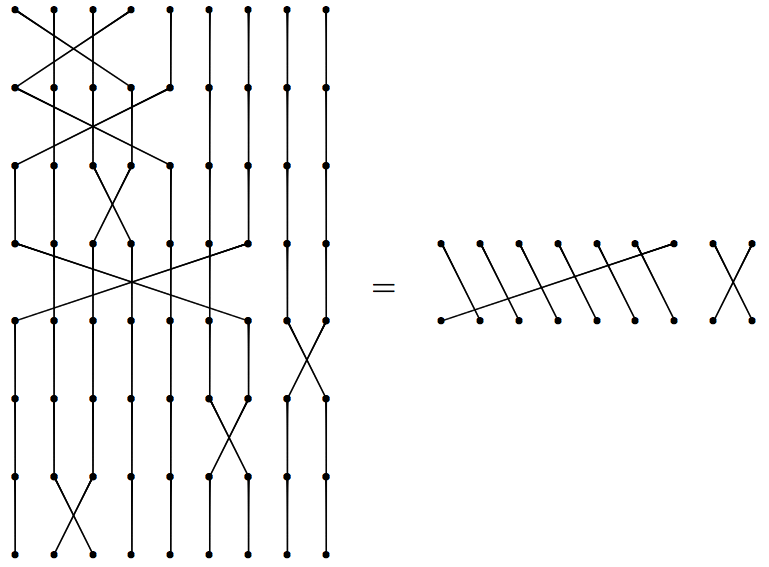## Rephrasing the problem

The problem can be restated as:

Can we multiply a permutation by a sequence of transpositions that we haven't used yet to obtain the identity permutation?

It turns out that, in general, the answer is yes, but we need to add in two more "clean" people to pull this off.

## The Futurama Theorem

Here is the solution presented in the show.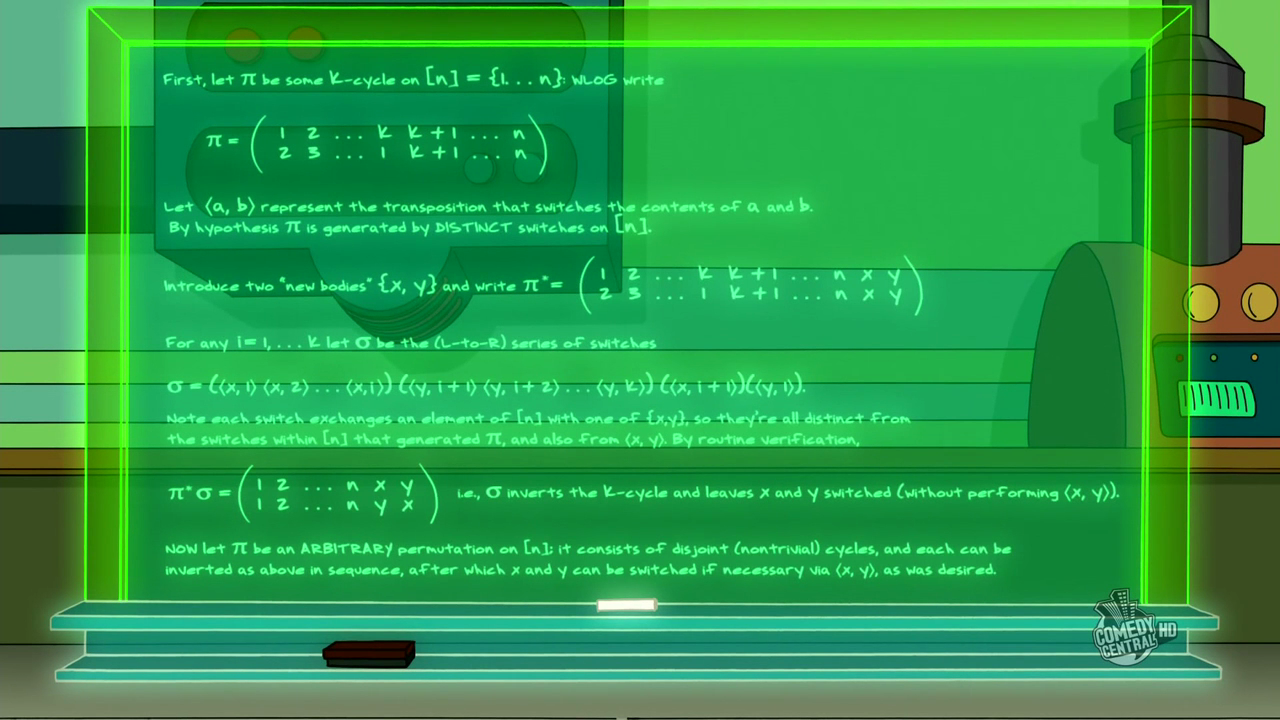Note:This image is taken from The Infosphere: The Futurama Wiki.

## Cycle notation

Before describing the solution, we need to introduce a more efficient way of encoding our permutations. One such method is called cycle notation.

### Example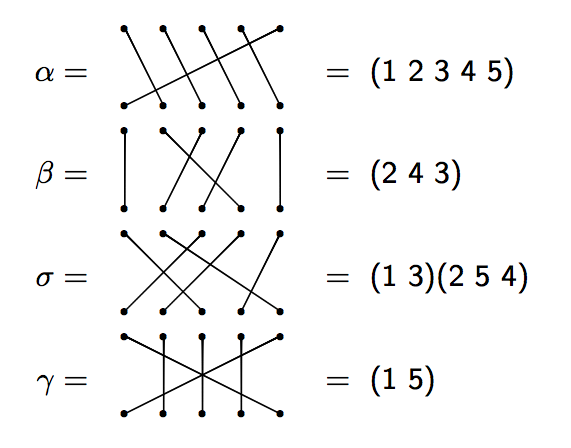## Cycle notation (continued)

Let's try multiplying using cycle notation. Note: We will multiply left to right. The more common way is to do it right to left (like function composition).

### Example

Consider $\alpha, \beta, \sigma$, and $\gamma$ in $S_{5}$ as in the previous examples. Then

$\alpha \beta=(1, 2, 3, 4, 5)(2, 4, 3)=(1, 4, 5).$

On the other hand,

$\beta \alpha=(2, 4, 3)(1, 2, 3, 4, 5)=(1, 2, 5).$

### Example

Let $\beta$ and $\gamma$ be as before. Then

$\gamma\beta =(2\ 4\ 3)(1\ 5)=(1\ 5)(2\ 4\ 3)=\beta\gamma.$

We saw earlier that $\beta$ and $\gamma$ commute with each other.

• In cycle notation, the Futurama permutation is $(1,2,3,4,5,6,7)(8,9)$.
• The interpretation of a cycle $(\ldots,i,j,\ldots)$ is that the body numbered $i$ passes its mind to the body $j$, etc.
• The inverse of a cycle is just the cycle written in the reverse order.
• Transpositions are of the form $(i,j)$, which means that $i$ and $j$ swap minds.
• "Disjoint" cycles (including transpositions) commute.
• If we can figure out how to "fix" one (disjoint) cycle at a time, then we can likely fix them all.

## Fixing a single 7-cycle

Consider just the 7-cycle $(1,2,3,4,5,6,7)$. Introduce two new bodies that have not had their minds swapped, say $x$ and $y$. To return all minds of 1-7 back to their rightful owner, multiply the cycle (on the right) by the following sequence of transpositions:

$(x,1)(y,7)(y,6)(y,5)(y,4)(y,3)(y,2)(y,1)(x,7)$

Let's verify that this actually works! First, notice that all of these transpositions are distinct and since $x$ and $y$ are new, we never used any of the transpositions to obtain our original scrambling of minds.

$(1,2,3,4,5,6,7)(x,1)(y,7)(y,6)(y,5)(y,4)(y,3)(y,2)(y,1)(x,7)=???$

Note: $x$ and $y$ now have their minds swapped with each other, but they never sat in the machine at the same time!

## Fixing an arbitrary $k$-cycle

Consider the $k$-cycle $(1,2,\ldots,k)$. How do we "fix" this cycle?

As before, introduce two new bodies that have not had their minds swapped, say $x$ and $y$. To fix a $k$-cycle, multiply the cycle (on the right) by the following:

$(x,1)(y,k)(y,k-1)\cdots (y,2)(y,1)(x,k)$

Why does this work?!

• First observation: $(1,2,\ldots,k)(x,1)(y,k)=(1,2,\ldots,k-1,y,k,x)$.
• Then $(y,k-1)$ puts the mind of $k-1$ back where it belongs.
• And then $(y,k-2)$ puts the mind of $k-2$ back where it belongs.
• We continue this way and in the second to last step, $(y,1)$ puts the mind of 1 back where it belongs.
• And finally, we finish by swapping $x$ and $k$.
• We now have $x$ and $y$ with swapped minds (but they have never swapped with each other).

## Fixing products of disjoint cycles

What do we do when we have multiple cycles to start with?

• Use $x$ and $y$ to fix each individual cycle.
• When doing this, $x$ and $y$ will sometimes be swapped.
• If there is an even number of disjoint cycles, then we're done after we fix each individual cycle.
• If there is an odd number of disjoint cycles, then after fixing each individual cycle, finish by swapping $x$ and $y$.

## Let's try it out!

• We need some volunteers! Pick two people to be $x$ and $y$.
• Write your name on a name tag and on a sheet of paper. The name tag represents your body and the sheet of paper represents your mind.
• Now, randomly shuffle your sheets of paper (i.e., minds) amongst each other, but $x$ and $y$ should not swap. Other than $x$ and $y$, each person should have exactly one mind that does not belong to them.
• Determine the disjoint cycles. Hint: If someone is holding your mind, stand next to them with that person on your right.
• Number yourselves in a way that makes sense. Put these numbers on your name tag and your sheet of paper.
• Now, see if you can apply our algorithm to return each mind to the appropriate person.

## Closing remarks

• Our algorithm/proof is a special case of the one given on the board in the TV episode.
• In the TV episode, $x$ and $y$ are Harlem Globetrotters.
• Applying our algorithm to the arrangement of minds in Futurama requires 13 moves.
• But since Fry and Zoiberg only swapped with each other and since there is only one other cycle, we could use Fry and Zoiberg as $x$ and $y$, respectively in our algorithm. This only requires 9 moves.

### Questions to ponder

• Can we do better (i.e., fewer moves to fix the cycles)?
• Under what circumstances do we not need to introduce two "clean" people?
• Would only using one "clean" person ever be useful?
• Could you use fewer moves if you allowed more than two "clean" people?

# Thank you!

### Dana C. Ernst Plymouth State University

These slides were made using deck.js and MathJax.

/

#